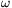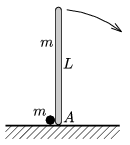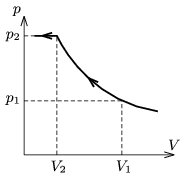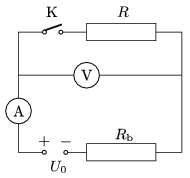Mathematical and Physical Journal
for High Schools
Issued by the MATFUND Foundation
 Already signed up? New to KöMaL?

# KöMaL Problems in Physics, March 2011

Show/hide problems of signs:## Problems with sign 'M'

Deadline expired on April 11, 2011.

M. 313. Measure the coefficient of friction between the tabletop and the body of a biro, which has a push button with a spring in it at one of its ends. Only tape measure is allowed to use.

(6 pont)

statistics## Problems with sign 'P'

Deadline expired on April 11, 2011.

P. 4326. A vessel, filled with water, is placed to a very accurate scale, and the reading on the scale is 308.48 g. A block which hangs on a spring balance is totally immersed into the water. Now the reading on the scale is 336.43 g. What is the reading on the spring balance if it was 1.75 N before the block was immersed into the water? What is the density of the block?

(3 pont)

solution (in Hungarian), statistics

P. 4327. One of the trains of the M2 metro line in Budapest consists of 5 cars. When the train starts, each car uses 300 A current from the 800 V voltage supply. How much does it cost to accelerate this train for 10 seconds, if the price of 1 kWh is 18 euro cents?

(3 pont)

solution (in Hungarian), statistics

P. 4328. When will the electricity bill of the Vízművek (Hungarian public utility company providing water) be greater, if the same amount of water flows from the tap at the groundfloor or at the tenth-floor?

(4 pont)

solution (in Hungarian), statistics

P. 4329. The jib of a tower crane is L=40 m long and it rotates about one of its ends in a horizontal plane at a constant angular speed of=0.03 s-1. On the jib a trolley moves away from the fixed end of the jib at a speed of u=0.08 m/s, with respect to the jib.

a) Determine the speed of the trolley with respect to the ground as a function of time.

b) Graph the path of the trolley with respect to the ground as it moves along the jib.

c) Determine the velocity of the trolley with respect to the ground as a function of time.

(4 pont)

solution (in Hungarian), statistics

P. 4330. A thin, L=2 m long rod of mass m is standing vertically on a horizontal frictionless plane. Next to its bottom endpoint A there is a pointlike object of mass m at rest. The rod fells out of its unstable equilibrium position, such that the paths of the small body and the rod are always in the same plane. What is the greatest speed of the small body?(6 pont)

solution (in Hungarian), statistics

P. 4331. One third of the space in a hollow sphere of inner diameter 8 cm is filled with water. The sphere is floating freely in a space station. What is the position of the water if the material of the sphere is

a) glass, to which water totally adheres,

b) silver, and the contact angle between the silver and the water is 90o?

(5 pont)

solution (in Hungarian), statistics

P. 4332. Two balls, made of soft copper alloy of specific heat capacity 400 J/(kg.K), collide head on. The initial speeds of the two balls, A and B, were the same and oppositely directed. After the collision ball A stops and ball B bounces back with the same speed as it had before the collision. Because of the collision the temperature of the balls increased withC. At least what was the speed of the balls before the collision?

(4 pont)

solution (in Hungarian), statistics

P. 4333. Is it possible that there exists a real gas, such that one of its isotherm intersects one of its adiabat at several different points on thediagram?

(5 pont)

solution (in Hungarian), statistics

P. 4334. In a container which is equipped with a piston there is a mixture of Oxygen and Nitrogen gas at a temperature of T=77.4 K. Keeping the temperature constant the mixture is slowly compressed. The pressure of the mixture as a function of the volume changes as shown in the figure, where V1=15 dm3 és p1=56.3 kPa.

a) How much Oxygen and Nitrogen are there in the container?

b) Determine thecoordinates of that single point on the isotherm, where the graph has a cusp.(5 pont)

solution (in Hungarian), statistics

P. 4335. The electromotive force of the voltage supply in the circuit, shown in the figure, is U0=9 V and its internal resistance Rb is unknown. The resistances of the meters and the resistor are also unknown. When the switch is closed then the measured current increases to twice of the previous value, and the reading on the voltmeter decreases to half of the original value.

a) By what factor is the resistance of the voltmeter greater than that of the resistor?

b) What are the readings on the voltmeter before and after closing the switch?(4 pont)

solution (in Hungarian), statistics

P. 4336. An electron of negligible initial speed is accelerated through a potential difference of a million volt. To what final speed will it accelerate, by what factor does its mass increase and what will its de Broglie wavelength be?

(4 pont)

solution (in Hungarian), statistics